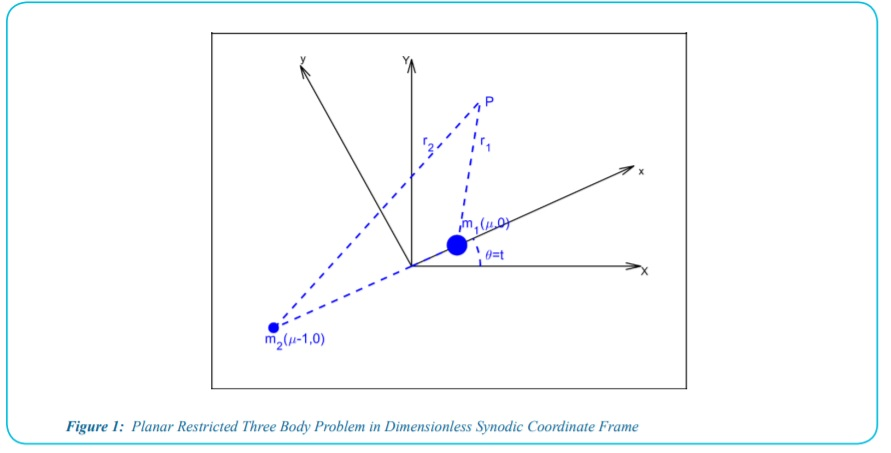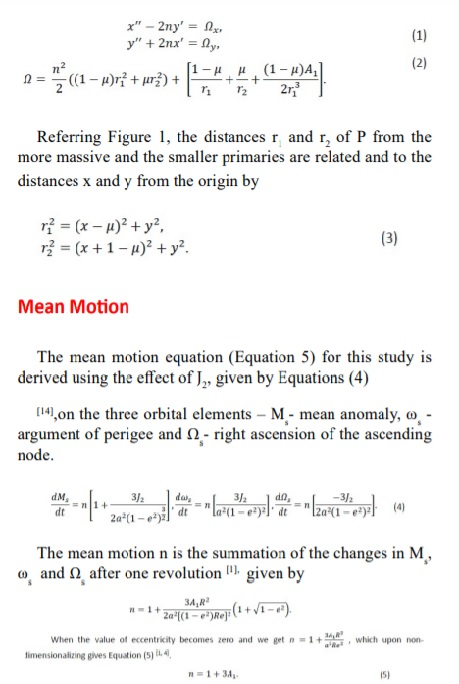## A note on commensurability induced by oblateness at L3

Arantza Jency A, Ram Krishan Sharma*

Citation: : Arantza Jency A, Ram Krishan Sharma”A note on commensurability induced by oblateness at L3”. American Research Journal of Physics, vol 6, no. 1, 2020, pp. 1-4.

Copyright Ram Krishan Sharma, Et al. This is an open access article distributed under the Creative Commons Attribution License, which permits unrestricted use, distribution, and reproduction in any medium, provided the original work is properly cited.

## Abstract:

It is known that there exists a near one-to-one commensurability ratio between the planar angular frequencies (s1,2,3) and the corresponding angular frequency (sz) in the z-direction at the three collinear points (L1,2,3) in the three-dimensional restricted three-body problem. It is significant for small and practically important values of the mass parameter (µ). In this note we have used a new mean motion expression which includes the secular effects of oblateness on argument of perigee, right ascension of ascending node and mean anomaly, which was used in the studies[2,3,4,5]. We establish that there is ono-to-one commensurability at the external points L3 (to the right of the more massive primary), when the more massive primary is considered as an oblate spheroid with its equatorial plane coincident with the plane of motion. This study will be useful in generating the halo orbits at L3. For Saturn-Titan system, the values of the µ and oblateness coefficient (A1) are 0.000236695 and 0.000039653936, respectively .It is interesting to note that the value of A1 obtained for one-to-one commensurability is 0.0000344978 for this system, which is very close to the actual value. Thus, halo orbits of small size can be generated in Saturn-Titan system at L3.

## Description:

#### Introduction

The locations of the Lagrangian points in the restricted three-body problem (CRTBP) by assuming both the primaries as oblate spheroids with their equatorial planes coincident with the plane of motion was calculated in . In  the location of the collinear points in the same problem was studied numerically for some systems of astronomical interest. These equilibria were shown to be unstable in general, though the existence of conditional infinitesimal (linearized) periodic orbits around them was established. However, the secular effect of oblateness of the primaries on the motion of the primaries was not included. Later the oblateness of only the more massive primary was considered and the secular effect of oblateness  on the mean motion of the primaries was included in [9, 10, 11]. In [9, 10], the critical mass value µc was found to decrease with oblateness. In [10, 11], a numerical investigation of the locations of the five equilibrium points was made for some systems of astronomical interest. Periodic solutions of the linearized equations of motion around the five equilibrium points were studied. The angular frequency in the z-direction (sz) was found to be more than the mean motion n. In  it was established that the oblateness induces a one-to-one commensurability at the exterior point L3 and at the interior point L2 for 0 ≤ µ ≤ ½ and at L1 no such commensurability exits. L2 Series expansions were found for the long-periodic (s4) short-periodic (s5) orbits. s4 was found to increase and s5 was found to decrease with oblateness.

In this paper we have included the secular effect of oblateness on the mean anomaly, argument of perigee and right ascension of ascending node . We have utilized the new mean motion to study the locations of the collinear equilibrium points. We have proved the existence of one-to-one commensurability ratio between the planar angular frequencies (s3 ) and the corresponding angular frequency (sz) in the z-direction at the collinear point (L3).

#### Equations of Motion

The problem is defined in the non-dimensional pulsating synodic coordinate frame as given by Figure 1. The barycentre of the primaries mark the origin of the system which rotates about the z-axis (perpendicular to the plane of motion of primaries. The mass ratio is the ratio of the mass of less massive primary m2 to the sum of the masses of the primaries m1 + m2) which is unity in the non-dimensional system. Point represents the point mass (with infinitesimal mass).

The equations of motion in terms of the dimensionlessframe is given by Equations (1)[11, 13]. The forcefunction Ω [9, 11] in the equations of motion is given by Equation (2). The oblateness of the more massive primary A1 = (AE2-AP2)/5R2, AE and AP are equatorial and polar radii, respectively, and R is the distance between them, affects the force function of the system.#### Location of Collinear Equilibrium Points

The equations of motion (1) are found to have singular solutions at five points  called the Lagrange points, liberation points or equilibrium points. Three of these equilibrium points (collinear equilibrium points -

## References

1. Sharma RK, Sellamuthu, H and Jency AA. Perturbed Trojan dynamics in the solar system, AAS AIAA Astrodynamics Specialist Conference AAS 20-704, August 2020. Available from: https://www.researchgate.net/ publication/343547691.

2. Johnson A, Sharma RK. Locations of Lagrangian points and periodic orbits around triangular points in the photo gravitational elliptic restricted three-body problem with oblateness. International Journal of Advanced Astronomy. 2019;7(2):25–38. doi:10.14419/ijaa.v7i2.29377.

3. Jency AA, Sharma RK. Location and stability of the triangular Lagrange points in photo-gravitational elliptic restricted three body problem with the more massive primary as an oblate spheroid. International Journal of Advanced Astronomy. 2019;7(2):57–62. DOI:10.14419/ijaa.v7i2.29814

4. Arohan R, Sharma RK. Periodic orbits in the planar restricted photogravitational problem when the smaller primary is an oblate spheroid. Indian Journal of Science and Technology. 2020; 13(16): 1630- 1640. https://doi.org/10.17485/IJST/v13i16.401

5. Jency AA, Sharma RK, Gaagandeep Singh. Stationary solutions, critical mass, Tadpole orbits in the circular restricted three-body problem with the more massive primary as an oblate spheroid. Indian Journal of Science and Technology (Accepted for publication, October 2020).

6. Sharma RK. Perturbations of Lagrangian points in the restricted threebody problem. Indian Journal of Pure and Applied Mathematics, 1975; 6:1099-1102.

7. Sharma RK, Subba Rao PV. Collinear equilibria and their characteristics exponents in the restricted three- body problem when the primaries are oblate spheroids. Celestial Mechanics, 1975; 12,189-201. https://doi.org/10.1007/BF01230211.

8. McCuskey SW. Introduction to Celestial Mechanics. Addison-Wesley Publishing Company. 1963

9. Subba Rao PV, Sharma RK. A note on the stability of the triangular points of equilibrium in the restricted three-body problem. Astronomy and Astrophysics. 1975; 43:381–383. Available from: http://adsabs.harvard. edu/abs/1975A%26A43..381S.

10. Sharma R. K. Study of Periodic Orbits in Restricted Problem taking the Bigger Primary an Oblate Spheroid. Ph.D. Thesis. University of Delhi. 1976

11. Sharma RK, Subba Rao PV. Stationary solutions and their characteristic exponents in the restricted three-body problem when the more massive primary is an oblate spheroid. Celestial Mechanics. 1976; 13:137–149. https://doi.org/10.1007/BF01232721.

12. Sharma RK, Subba Rao PV. A case of commensurability induced by oblateness. Celestial Mechanics. 1978: 18,185-194. Available from: adsabs.harvard.edu › full › 1978CeMec..18..185S

13. Szebehely V, and. Theory of Orbits. Academic Press: New York, 1967. eBook ISBN: 9780323143462.

14. Curtis HD. Orbital Mechanics for Engineering Students. Elsevier: Waltham, 2014. ISBN-13: 978- 0080977478.